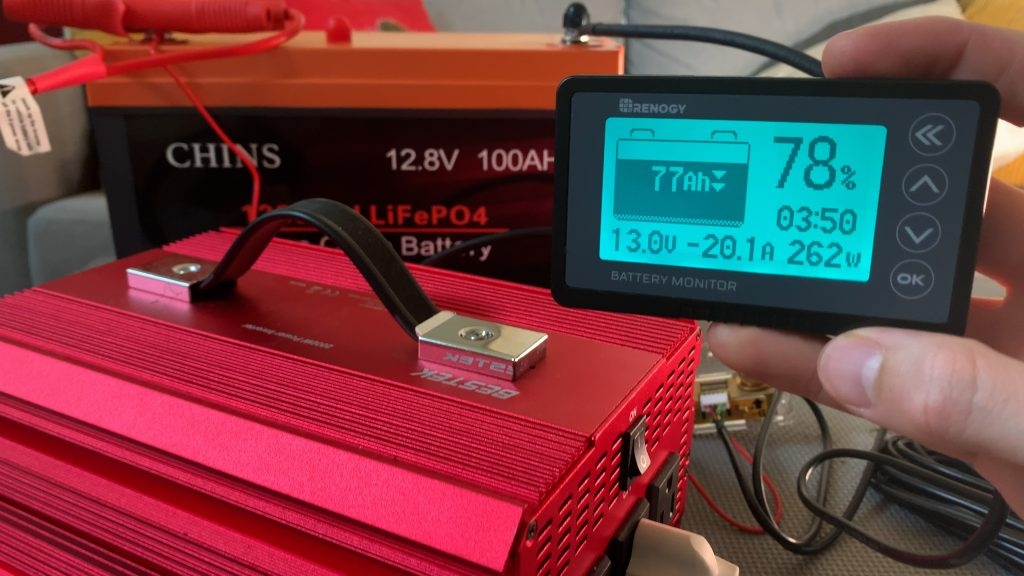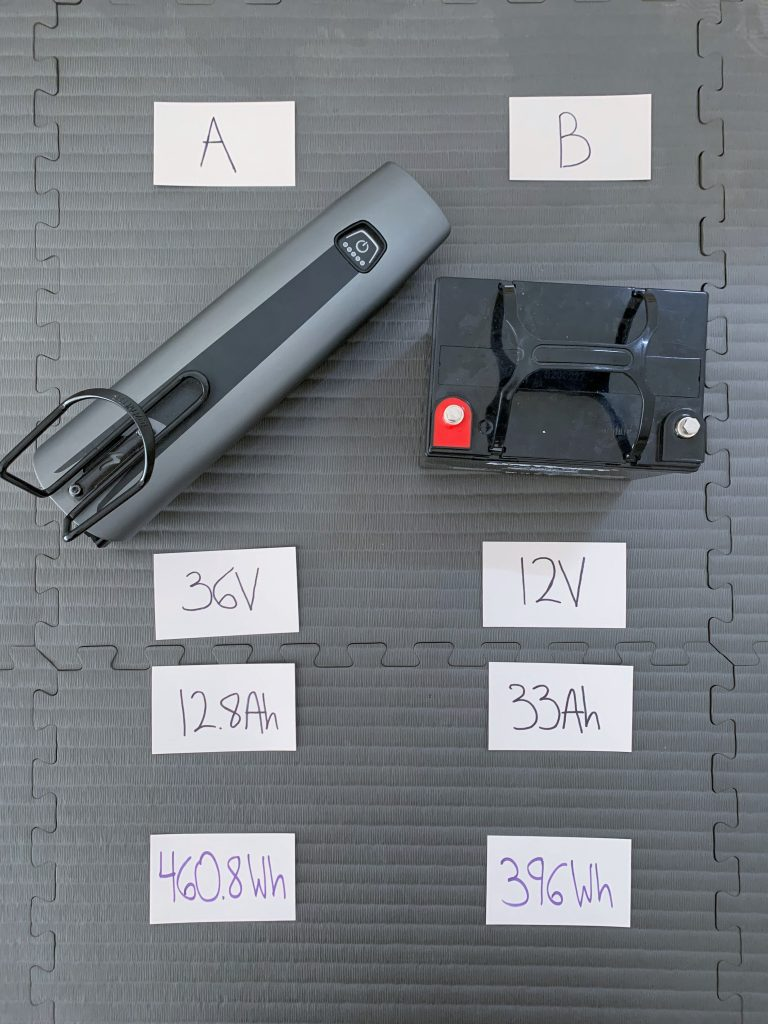# Amps to Amp Hours (A to Ah) Conversion Calculator

Use our interactive calculator to easily convert amps to amp hours (A to Ah).

Error: This field is required.
Error: This field is required.
amp hours
Suggested battery:
Note: Based on your inputs, it looks like this battery (or batteries) has enough usable capacity to meet your energy needs. But check that it meets all your other requirements before going with this option.
Conversion formula: Ah = A × hrs

Or would you rather convert amp hours to amps?

## Amps to Amp Hours Conversion Chart

Here’s a conversion chart converting common values of amps to amp hours, over a duration of 1 hour and 1 day.

## What Is an Amp Hour?

An ampere hour (Ah) — often shortened to amp hour — is a unit of electric charge commonly used to measure the capacity of a battery.

It represents the amount of charge that can be delivered by a battery at a constant rate of 1 amp for 1 hour. In other words, a battery’s amp hour rating is a measure of the total amount of electrical energy that a battery can store and provide over a specific period.

For instance, this 12V lithium battery I own has a rating of 100 amp hours. (A battery’s amp hour rating is often referred to as its “capacity.”)

This rating tells me that this battery can output 1 amp for 100 hours, or 2 amps for 50 hours, or 10 amps for 10 hours, and so on (not taking into account the Peukert effect).

You will also often see phones and portable power banks use milliamp hours (mAh) to express their battery capacity. 1 amp hour is equal to 1,000 milliamp hours.

### Why Convert Amps to Amp Hours?This battery monitor shows that the 100Ah LiFePO4 battery it’s connected to is being drained at a rate of around 20 amps. At that rate, the battery will last around 5 hours.

Converting amps to amp hours is a good starting point when estimating how much battery capacity you need. For instance, if you’re considering buying a 12V 100Ah lithium battery, then you know it has a capacity of 100 amp hours.

So if you have a 12V electric cooler that consumes 4 amps on average, then a 12V 100Ah lithium battery can run the cooler for around 25 hours on a full charge.

Alternatively, you can use amp hours to express a device’s energy consumption over time. If a light uses 1 amp and runs for 10 hours, then it has consumed 10 amp hours. If it runs for 20 hours, it has consumed 20 amp hours.

However, amp hours have a notable limitation: they don’t take into account voltage. So, if you’re ever comparing 2 batteries or estimating how long a battery can run a device, make sure everything has the same voltage.

If it doesn’t, you’ll need to convert amp hours to watt hours.

## Amp Hours vs Watt HoursComparing the amp hours and watt hours of 2 different batteries. Even though Battery A has fewer amp hours, when we take into account voltage by calculating watt hours, we can see that it actually holds more energy.

Watt hours are a better way to express how much capacity a battery has, or how much energy a device has consumed, because they take into account both amp hours and voltage.

To calculate watt hours, simply multiply amp hours times volts.

Formula: watt hours = amp hours × volts

Abbreviated formula: Wh = Ah × V

Calculator: Amp Hours to Watt Hours Calculator

Calculating watt hours is necessary to do whenever 2 batteries or devices have different voltages. Only use amp hours to estimate battery runtime or compare battery capacities when all the batteries and loads have identical voltages.

## How to Convert Amps to Amp Hours (A to Ah)

To convert amps to amp hours, simply multiply amps times hours.

Formula: amp hours = amps × hours

Abbreviated formula: Ah = A × hrs

### Example

Let’s say you have a 12V fan that you want to power with a 12V battery. You look at the fan’s electrical specifications and see that is uses 2 amps. You want to run the fan for around 3 hours a day.

To find out how many amp hours the fan uses per day, you’d do the following calculation:

`2 A × 3 hrs = 6 Ah`

Let’s go on to say that you want the battery to last for 5 days of use. In that case, you’d multiply the fans daily amp hour consumption by the number of days you’ll be running it off the battery.

`6 Ah × 5 days = 30 Ah`

Now you know that you need a 12V battery with at least 30 amp hours of usable capacity in order to be able to run your 12V fan for as long as you’d like.

## How to Convert Amp Hours to Amps (Ah to A)

To convert amp hours to amps, divide amp hours by hours.

Formula: amps = amp hours ÷ hours

Abbreviated formula: A = Ah ÷ hrs

### Example

Converting amp hours to amps can be helpful when estimating a device’s average power consumption. For example, when I tested how long a 12V 100Ah battery would run a 12V mini fridge, I tracked the fridge’s power consumption over time with a battery monitor.

The problem is that a fridge’s power consumption can vary widely based on if it’s actively cooling or not. In my test, my fridge’s power draw ranged from 0 to 4 amps at any given time. But how many amps was it using on average?

To find out, I needed to take the battery’s measured amp hour capacity (102Ah) as recorded by my battery monitor and divide it by the fridge’s runtime (102.5 hours).

`102Ah ÷ 102.5 hrs = 0.995 amps`

So, my 12V mini fridge was using about 1 amp on average during my test.

## More Electricity Calculators